PSABM6VY - Student Facing Task---Acc7.5 Lesson 2 Representing Proportional Relationships (7.RP.A.2, 8.EE.B, 8.EE.B.5)
Part A)

Here is a graph that could represent a variety of different situations.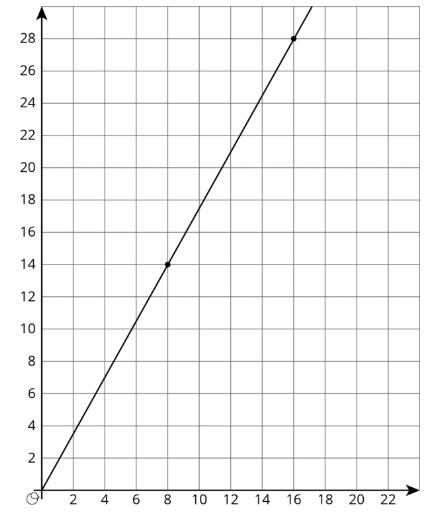Write an equation for the graph.

Write the equation using the "WIRIS editor" button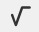Part B)

Sketch a new graph of this relationship.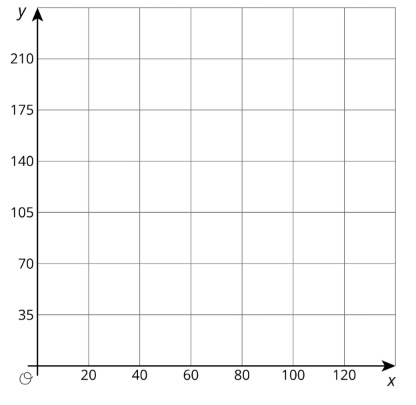Draw your graph on paper, take a picture, and upload it using the image upload icon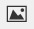If you do not have the ability to upload an image of your work type "Graph is on paper."

Part A)

Two large water tanks are filling with water. Tank A is not filled at a constant rate, and the relationship between its volume of water and time is graphed on each set of axes. Tank B is filled at a constant rateof  liters per minute. The relationship between its volume of water and time can be described by the equation, where t is the time in minutes and v is the total volume in liters of water in the tank.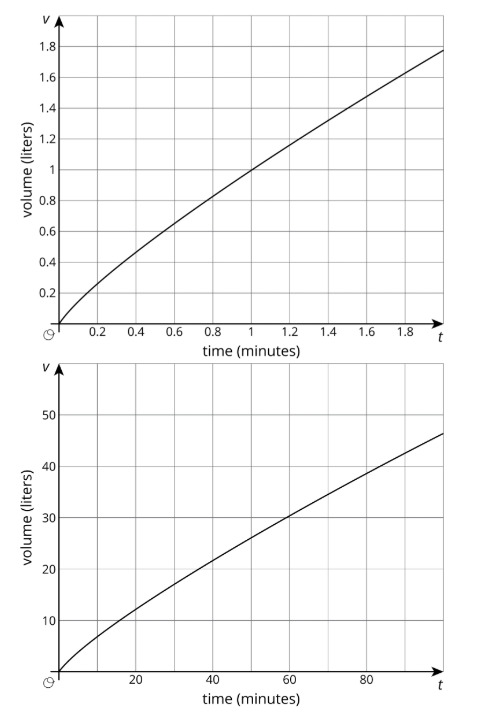Sketch and label a graph of the relationship between the volume of water v and time t for Tank B on each of the axes.

Draw your graph on paper, take a picture, and upload it using the image upload iconIf you do not have the ability to upload an image of your work type "Graph is on paper."

Part B)

After 30 seconds, which tank has the most water?

Select one:
Part C)

At approximately what times do both tanks have the same amount of water?

Part D)

At approximately what times do both tanks contain 1 liter of water?

Part E)

At approximately what times do both tanks contain 20 liters of water?

Part A)

A giant tortoise travels at 0.17 miles per hour and an arctic hare travels at 37 miles per hour.

Draw separate graphs that show the relationship between time elapsed, in hours, and distance traveled, in miles, for both the tortoise and the hare.

Draw your graph on paper, take a picture, and upload it using the image upload iconIf you do not have the ability to upload an image of your work type "Graph is on paper."

Part B)

Would it be helpful to try to put both graphs on the same pair of axes? Why or why not?

Part C)

The tortoise and the hare start out together and after half an hour the hare stops to take a rest. How long does it take the tortoise to catch up?

Part A)

Here are two ways to represent a situation.

Description: Jada and Noah counted the number of steps they took to walk a set distance. To walk the same distance,

• Noah took 10 steps

Then they found that when Noah took 15 steps, Jada took 12 steps.

Equation: Let x represent the number of steps Jada takes and let y represent the number of steps Noah takes.Create a table that represents this situation with at least 3 pairs of values.

Draw your graph on paper, take a picture, and upload it using the image upload icon.

If you do not have the ability to upload an image of your work type "Graph is on paper."

Part B)

Graph this relationship and label the axes.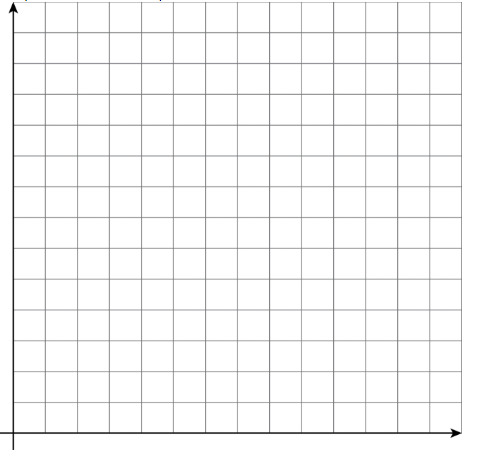Draw your graph on paper, take a picture, and upload it using the image upload icon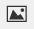If you do not have the ability to upload an image of your work type "Graph is on paper."

Part C)

How can you see or calculate the constant of proportionality in each representation? What does it mean?

Part D)

Explain how you can tell that the equation, description, graph, and table all represent the same situation.

Part E)

Here are two ways to represent a situation.

Description: The Origami Club is doing a car wash fundraiser to raise money for a trip. They charge the same price for every car. After 11 cars, they raised a total of $93.50. After 23 cars, they raised a total of$195.50.

Table: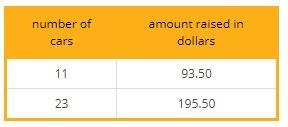Write an equation that represents this situation. (Use c to represent number of cars and use m to represent amount raised in dollars.)

Write the equation using the "WIRIS editor" buttonPart F)

Create a graph that represents this situation.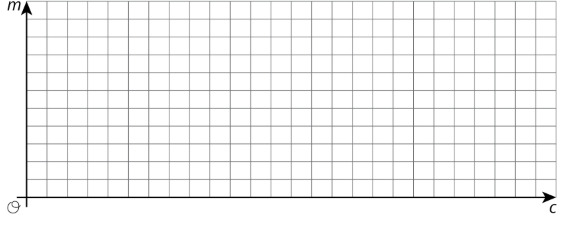Draw your graph on paper, take a picture, and upload it using the image upload iconIf you do not have the ability to upload an image of your work type "Graph is on paper."

Part G)

How can you see or calculate the constant of proportionality in each representation? What does it mean?

Part H)

Explain how you can tell that the equation, description, graph, and table all represent the same situation.

Part A)

Ten people can dig five holes in three hours. If n people digging at the same rate dig m holes in d hours:

is n proportional to m when d=3?